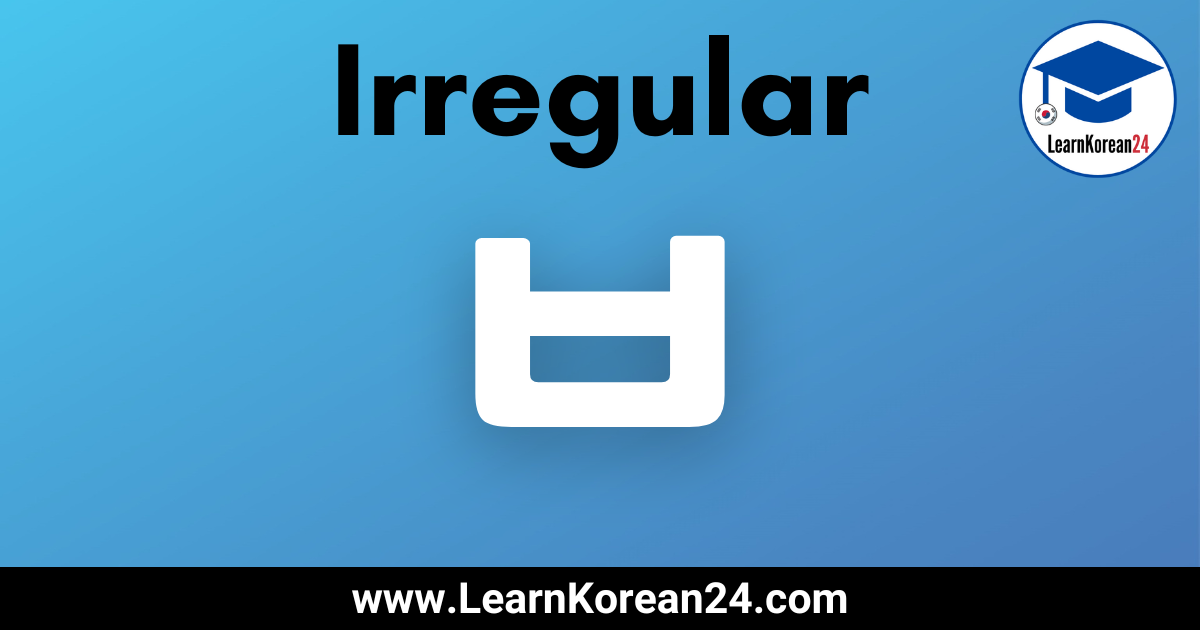# Korean Irregular Verbs – ㅂ Irregular (ㅂ 불규칙)Just like in other languages, there are many irregular verbs in Korean (불규칙 동사). One of the most common types of irregular verbs in Korean is ㅂ irregular verbs. An example of an irregular verb that you have probably heard of is 고맙다 (to be thankful).

What makes these verbs irregular is that they are not conjugated in the same way as regular verbs. So, for example, when 고맙다 is conjugated in the present tense, it becomes 고마워요 and not 고맙어요.

To help you learn about these irregular verbs, we have put together a list of irregular verbs with ㅂ (ㅂ 불규칙). You will also learn how to conjugate irregular verbs with ㅂ. Below you will also find a printable list of irregular verbs ( ㅂ 불규칙) and a printable conjugation chart to help you learn how to conjugate irregular ㅂ verbs.

## Korean ㅂ Irregular Verbs

There are many verbs in Korean that end in a that are irregular. However, not all verbs that end in are irregular. This makes it particularly important to learn which verbs are regular and which ones are irregular.

Here is a list of irregular Korean verbs that end in (ㅂ 불규칙):

• 덥다 = to be hot
• 맵다 = to be spicy
• 쉽다 = to be easy
• 춥다 = to be cold
• 귀엽다 = to be cute
• 가깝다 = to be close
• 가볍다 = to be light (weight)
• 고맙다 = to be thankful
• 무겁다 = to be heavy
• 무섭다 = to be scary
• 반갑다 = to be pleasing
• 어렵다 = to be difficult
• 시끄럽다 = to be noisy
• 아름답다 = to be beautiful
• 굽다 = to roast/bake
• 깁다 = to fix/mend
• 줍다 = to pick up
• 눕다 = to lie (down)
• 더럽다 = to be dirty
• 부럽다 = to be envious
• 어둡다 = to be dark
• 두렵다 = to be fearful
• 즐겁다 = to be enjoyable
• 새롭다 = to be new
• 외롭다 = to be lonely
• 이롭다 = to be benificial
• 까다롭다 = to be picky
• 흥미롭다 = to be interesting
• 밉다 = to be hateful
• 뜨겁다 = to be hot (to touch)
• 차갑다 = to be cold (to touch)
• 지혜롭다 = to be wise
• 돕다* = to help
• 곱다* = to be pretty

## How To Conjugate Irregular ㅂ Verbs

Learning how to conjugate irregular ㅂ verbs can be particularly confusing for Korean learners. This is because not only are irregular verbs conjugated differently, irregular verbs do not always behave irregularly.

The first thing to know about conjugating irregular ㅂ verbs is that irregular ㅂ verbs only behave irregularly if the ending which is attached to the verb starts with a vowel.

So, with verb endings such as ~(스)ㅂ니다, that start with a consonant, irregular verbs do not behave irregularly. Instead, they behave the same as regular verbs and are therefore conjugated the same as regular verbs.

For example, 고맙다 + ~(스)ㅂ니 = 고맙습니다. As you can see, the verb stem doesn’t change and it is conjugated the same as regular verbs.

However, if attaching an ending that starts with a vowel, irregular verbs behave irregularly and are conjugated differently.

For example, 고맙다 + 어요 = 고마워요 (not 고맙어요).

Let’s take a look at the conjugation rules for irregular ㅂ verbs.

### Irregular ㅂ Conjugation Rules

For almost all irregular verbs, this is the rule to follow when conjugating these verbs with endings that end in a vowel:

Drop ㅂ and add 우 before the verb ending.

For example, to conjugate the irregular verb 춥다 in the present tense, first drop the so you are left with . Then add and then add the verb ending 어요.

추 + 우 + 어요 = 추워요

Here are some examples of irregular ㅂ verbs conjugated in the present, past, and future tense.

*There are just 2 exceptions to the above conjugation rule. The verbs 돕다 (to help) and the verbs 곱다 (to be pretty) are conjugated differently with verb endings starting with 아/어/여. When these 2 verbs are conjugated with endings starting with 아/어/여, is added, not 우.

## Review

Of the 7 different types of irregular verbs, irregular verbs are perhaps the most confusing. However, over time, as you speak and learn more and more Korean, using these irregular verbs will become second nature.

Here are the most important things to remember about irregular ㅂ verbs:

1. Not all verbs that end in are irregular.
2. ㅂ irregular verbs only behave irregularly when you attach an ending which starts with a vowel. When attaching endings that start with a consonant, irregular verbs are conjugated in the same way as regular verbs.
3. The general rule to follow when conjugating irregular verbs is to drop ㅂ and add before the ending.
4. The only 2 exceptions to the above rule is when the verbs 돕다 or 곱다 are followed by an ending which starts with 아/어/여. In this case, is added, not .

## Irregular ㅂ Verbs – Conjugation Chart

To help you learn how to conjugate irregular ㅂ verbs, you can download and print this irregular verbs chart PDF. This chart includes some common irregular verbs with ㅂ and shows you how to conjugate these verbs with some common verb endings.

## More Korean Irregular Verb Lessons

There are seven types of irregular verbs in Korean. For more lessons on Korean Irregular verbs, click on the links below.